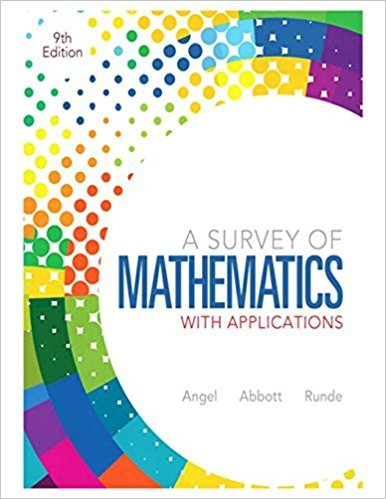×
×

# Solutions for Chapter 12.11: Probability## Full solutions for A Survey of Mathematics with Applications | 9th Edition

ISBN: 9780321759665Solutions for Chapter 12.11: Probability

Solutions for Chapter 12.11
4 5 0 374 Reviews
23
2
##### ISBN: 9780321759665

This expansive textbook survival guide covers the following chapters and their solutions. Chapter 12.11: Probability includes 25 full step-by-step solutions. Since 25 problems in chapter 12.11: Probability have been answered, more than 74916 students have viewed full step-by-step solutions from this chapter. A Survey of Mathematics with Applications was written by and is associated to the ISBN: 9780321759665. This textbook survival guide was created for the textbook: A Survey of Mathematics with Applications, edition: 9.

Key Math Terms and definitions covered in this textbook
• Back substitution.

Upper triangular systems are solved in reverse order Xn to Xl.

• Basis for V.

Independent vectors VI, ... , v d whose linear combinations give each vector in V as v = CIVI + ... + CdVd. V has many bases, each basis gives unique c's. A vector space has many bases!

• Characteristic equation det(A - AI) = O.

The n roots are the eigenvalues of A.

• Commuting matrices AB = BA.

If diagonalizable, they share n eigenvectors.

• Hessenberg matrix H.

Triangular matrix with one extra nonzero adjacent diagonal.

• Least squares solution X.

The vector x that minimizes the error lie 112 solves AT Ax = ATb. Then e = b - Ax is orthogonal to all columns of A.

• Left nullspace N (AT).

Nullspace of AT = "left nullspace" of A because y T A = OT.

• Matrix multiplication AB.

The i, j entry of AB is (row i of A)·(column j of B) = L aikbkj. By columns: Column j of AB = A times column j of B. By rows: row i of A multiplies B. Columns times rows: AB = sum of (column k)(row k). All these equivalent definitions come from the rule that A B times x equals A times B x .

• Minimal polynomial of A.

The lowest degree polynomial with meA) = zero matrix. This is peA) = det(A - AI) if no eigenvalues are repeated; always meA) divides peA).

• Network.

A directed graph that has constants Cl, ... , Cm associated with the edges.

• Partial pivoting.

In each column, choose the largest available pivot to control roundoff; all multipliers have leij I < 1. See condition number.

• Rank one matrix A = uvT f=. O.

Column and row spaces = lines cu and cv.

• Reduced row echelon form R = rref(A).

Pivots = 1; zeros above and below pivots; the r nonzero rows of R give a basis for the row space of A.

• Reflection matrix (Householder) Q = I -2uuT.

Unit vector u is reflected to Qu = -u. All x intheplanemirroruTx = o have Qx = x. Notice QT = Q-1 = Q.

• Row picture of Ax = b.

Each equation gives a plane in Rn; the planes intersect at x.

• Row space C (AT) = all combinations of rows of A.

Column vectors by convention.

• Schwarz inequality

Iv·wl < IIvll IIwll.Then IvTAwl2 < (vT Av)(wT Aw) for pos def A.

• Semidefinite matrix A.

(Positive) semidefinite: all x T Ax > 0, all A > 0; A = any RT R.

• Spanning set.

Combinations of VI, ... ,Vm fill the space. The columns of A span C (A)!

• Symmetric factorizations A = LDLT and A = QAQT.

Signs in A = signs in D.

×